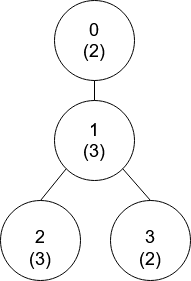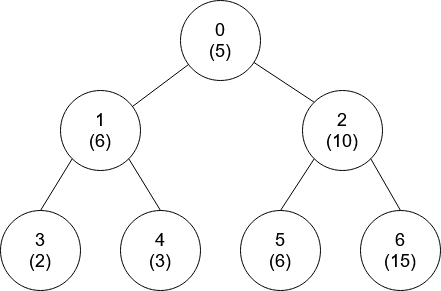# GeetCode Hub

There is a tree (i.e., a connected, undirected graph that has no cycles) consisting of `n` nodes numbered from `0` to `n - 1` and exactly `n - 1` edges. Each node has a value associated with it, and the root of the tree is node `0`.

To represent this tree, you are given an integer array `nums` and a 2D array `edges`. Each `nums[i]` represents the `ith` node's value, and each `edges[j] = [uj, vj]` represents an edge between nodes `uj` and `vj` in the tree.

Two values `x` and `y` are coprime if `gcd(x, y) == 1` where `gcd(x, y)` is the greatest common divisor of `x` and `y`.

An ancestor of a node `i` is any other node on the shortest path from node `i` to the root. A node is not considered an ancestor of itself.

Return an array `ans` of size `n`, where `ans[i]` is the closest ancestor to node `i` such that `nums[i]` and `nums[ans[i]]` are coprime, or `-1` if there is no such ancestor.

Example 1:```Input: nums = [2,3,3,2], edges = [[0,1],[1,2],[1,3]]
Output: [-1,0,0,1]
Explanation: In the above figure, each node's value is in parentheses.
- Node 0 has no coprime ancestors.
- Node 1 has only one ancestor, node 0. Their values are coprime (gcd(2,3) == 1).
- Node 2 has two ancestors, nodes 1 and 0. Node 1's value is not coprime (gcd(3,3) == 3), but node 0's
value is (gcd(2,3) == 1), so node 0 is the closest valid ancestor.
- Node 3 has two ancestors, nodes 1 and 0. It is coprime with node 1 (gcd(3,2) == 1), so node 1 is its
closest valid ancestor.
```

Example 2:```Input: nums = [5,6,10,2,3,6,15], edges = [[0,1],[0,2],[1,3],[1,4],[2,5],[2,6]]
Output: [-1,0,-1,0,0,0,-1]
```

Constraints:

• `nums.length == n`
• `1 <= nums[i] <= 50`
• `1 <= n <= 105`
• `edges.length == n - 1`
• `edges[j].length == 2`
• `0 <= uj, vj < n`
• `uj != vj`

class Solution { public int[] getCoprimes(int[] nums, int[][] edges) { } }